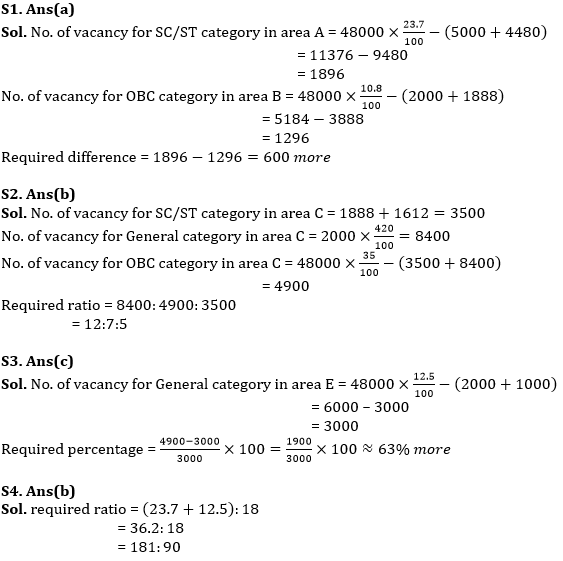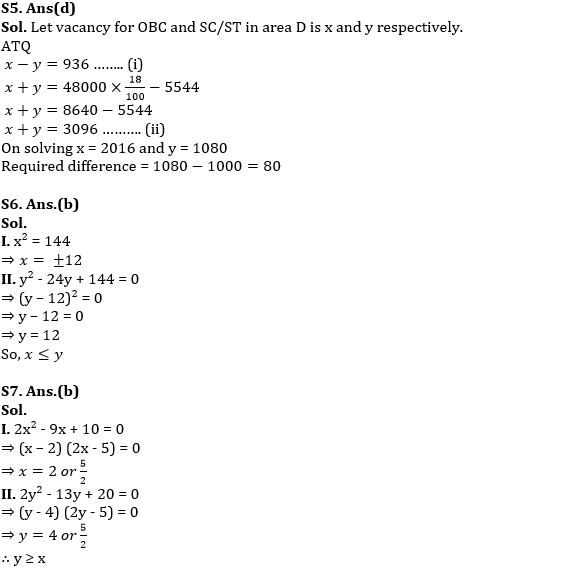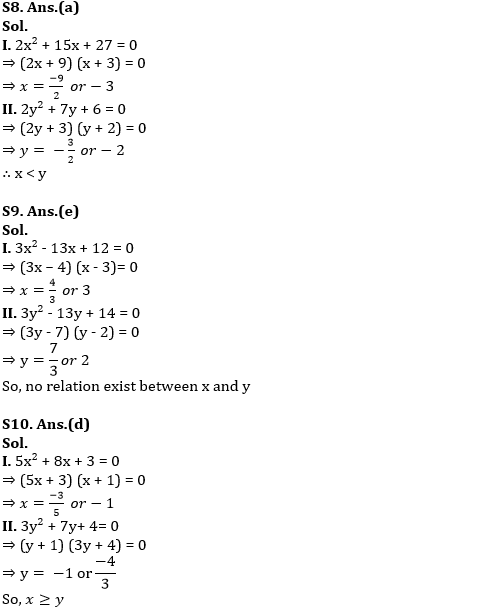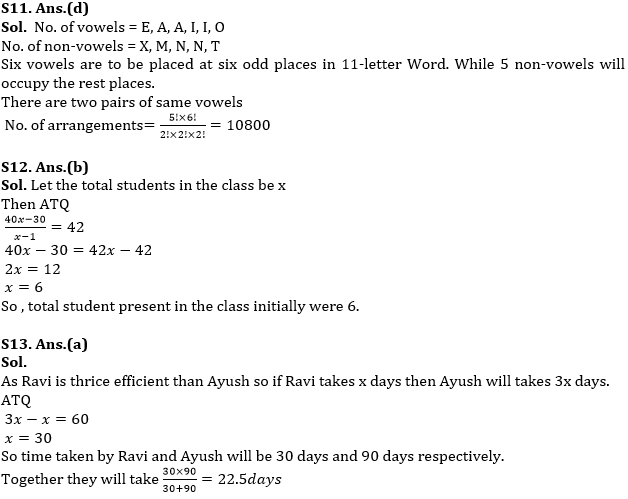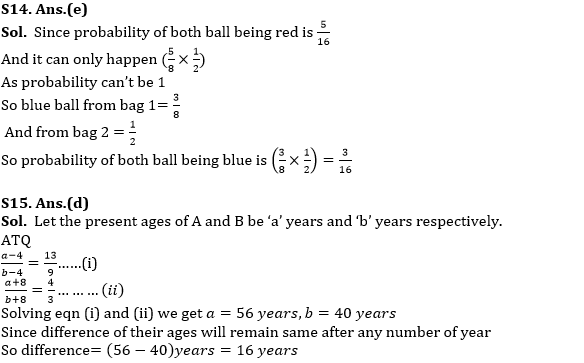Latest Banking jobs   »   Quantitative Aptitude Quiz For RBI Grade...

# Quantitative Aptitude Quiz For RBI Grade B/ ECGC PO/ SIDBI Grade A Prelims 2022- 28thApril

Directions (1-5): Pie chart given below shows percentage distribution of vacancy issued for the post of clerk by RRB in five different area and table shows distribution of no. of vacancy for three different categories i.e. (General, OBC, and SC/ST). Some data is missing in the table you have to calculate the data according to given information and answer the following questions.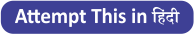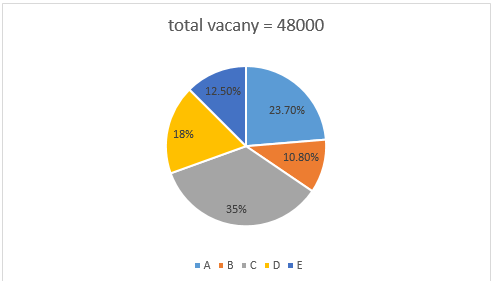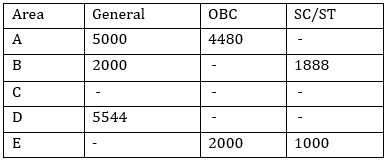Q1. Vacancy for SC/ST category in area A is how much more or less than no. of vacancy for OBC category in area B?
(a) 600 more
(b) 500 less
(c) 400 less
(d) 600 less
(e) 400 more

Q2. If in area C vacancy for General and SC/ST category is 320% more and 1612 more than vacancy of same category in area B respectively, find ratio of vacancy for General, OBC and SC/ST category in area C?
(a) 11:9:4
(b) 12:7:5
(c) 12:5:1
(d) 6:5:1
(e) None of these.

Q3. No. of vacancy for OBC category in area C is approximately how much percent more or less than no. of vacancy for General in area E? (use information of question no. 42)
(a) 63% less
(b) 60% less
(c) 63% more
(d) 67% more
(e) 68% less

Q4. What is the ratio of total vacancy in area A and E together to total vacancy in area D?
(a) 2:1
(b) 181:90
(c) 161:90
(d) 4:5
(e) 121:70

Q5. If in area D, difference between vacancy for OBC and SC/ST (OBC>SC/ST) is 936, then find vacancy for SC/ST in area D is how much more than vacancy for same category in area E?
(a) 100
(b) 108
(c) 64
(d) 80
(e) 120

Directions (6-10): In the following questions two equations numbered I and II are given. You have to solve both the equations and give answer
(a) If x < y
(b) If x ≤ y
(c) If y < x
(d) If y ≤ x
(e) If x =y or if no relationship can be established.

Q6. I. x² = 144
II. y² – 24y + 144 = 0

Q7. I. 2x² – 9x + 10 = 0
II. 2y² – 13y + 20 = 0

Q8. I. 2x² + 15x + 27 = 0
II. 2y² + 7y + 6 = 0

Q9. I. 3x² – 13x + 12 = 0
II. 3y² – 13y + 14 = 0

Q10. I. 5x² + 8x + 3 = 0
II. 3y² + 7y + 4 = 0

Q11.How many arrangements can be formed out of the letters of the word ‘EXAMINATION’ so that vowels always occupy odd places?
(a) 21600
(b) none of these
(c) 32400
(d) 10800
(e)5400

Q12. The average age of the students in a class is 40yrs and if a student whose age was 30 yrs is absent from the class, then average age of those student who were present goes up by 2. How many students were there in the class initially?
(a) 10
(b) 6
(c) 8
(d) 12
(e)15

Q13.RAVI KANT SHARMA is thrice as efficient as Ayush and hence completes a work in 60 days less than the numbers of days taken by Ayush to complete the work. What will be the number of days taken by both of them to complete the work together?(in days)
(a) 22.5
(b) 30
(c) 60
(d) 37.5
(e)45

Q14. Maanik has. a total of 20 red and blue marbles in two bags (each bag has marbles of both colors). A marble is randomly drawn from the first bag followed by another randomly drawn from the second bag, the probability of both being red is 5/16. What is the probability of both marbles being blue?
(a) 5/16
(b) 7/18
(c) none of these
(d) can’t be determined
(e)3/16

Q15. Four year ago, the ratio of the ages of A and B was 13:9 and eight year hence it would be 4:3. The difference of their ages four year hence will be?(in years)
(a) 15
(b) 10
(c) 12
(d) 16
(e)20

Solutions